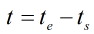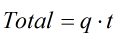# C203 – Flow rate Totalisation Check

## Description

This calculation is used for a totalisation check, flow rates are integrated with respect to time.

Kelton calculation reference C203

## Options

### Elapsed Time

• Duration
• Start and end time

Select a method of entering the elapsed.

### Flow rates

Flow rates q can be entered for any of the following quantities

• Mass
• Observed volume
• Standard volume
• Normal volume
• Energy

### Totals

• Use start and end totals

This option is used to input 2 totals and find the incremental total between the start and end totals.

## Calculations

### Calculate the elapsed time

If start and end time is selected for the time input:Where te = The time at the end of the test ts = The time at the start of the test

### Calculate the total

The total is calculated by multiplying the flow rate by the durationWhere t = Duration q = Flow rate

Note: The units will correspond to the flow rate, for example a mass value will be returned for a mass flow rate and a volume returned for a volume flow rate.

Back